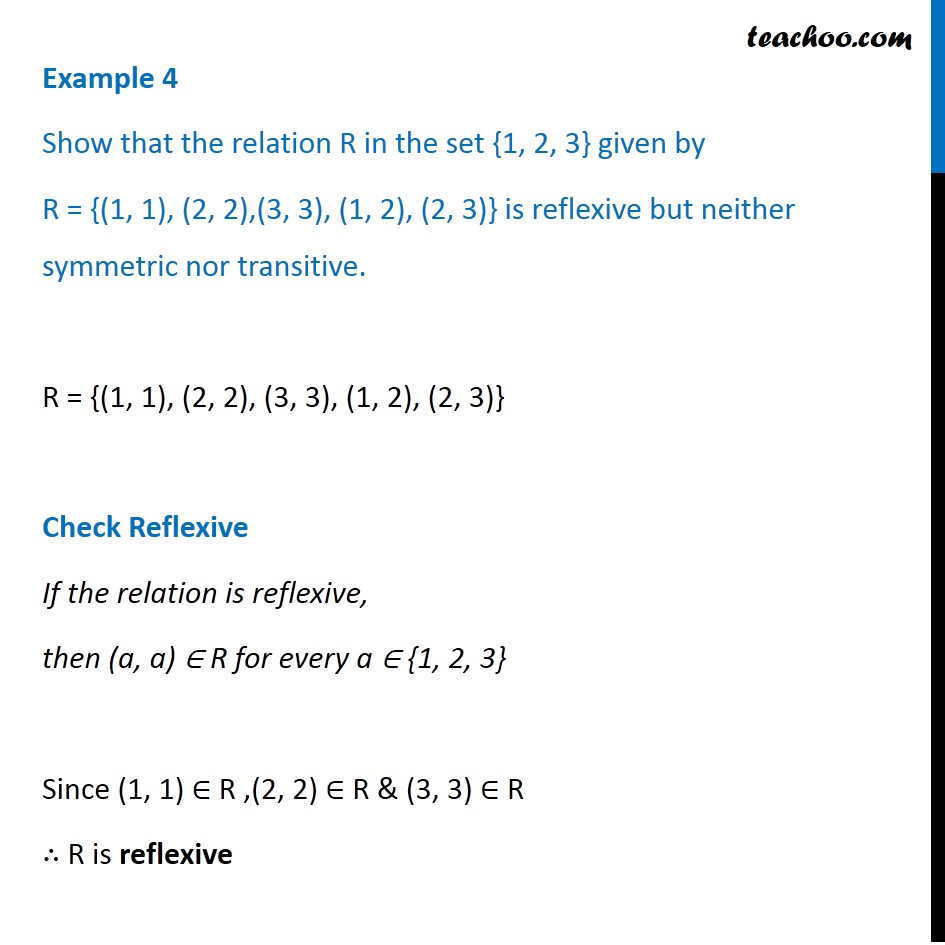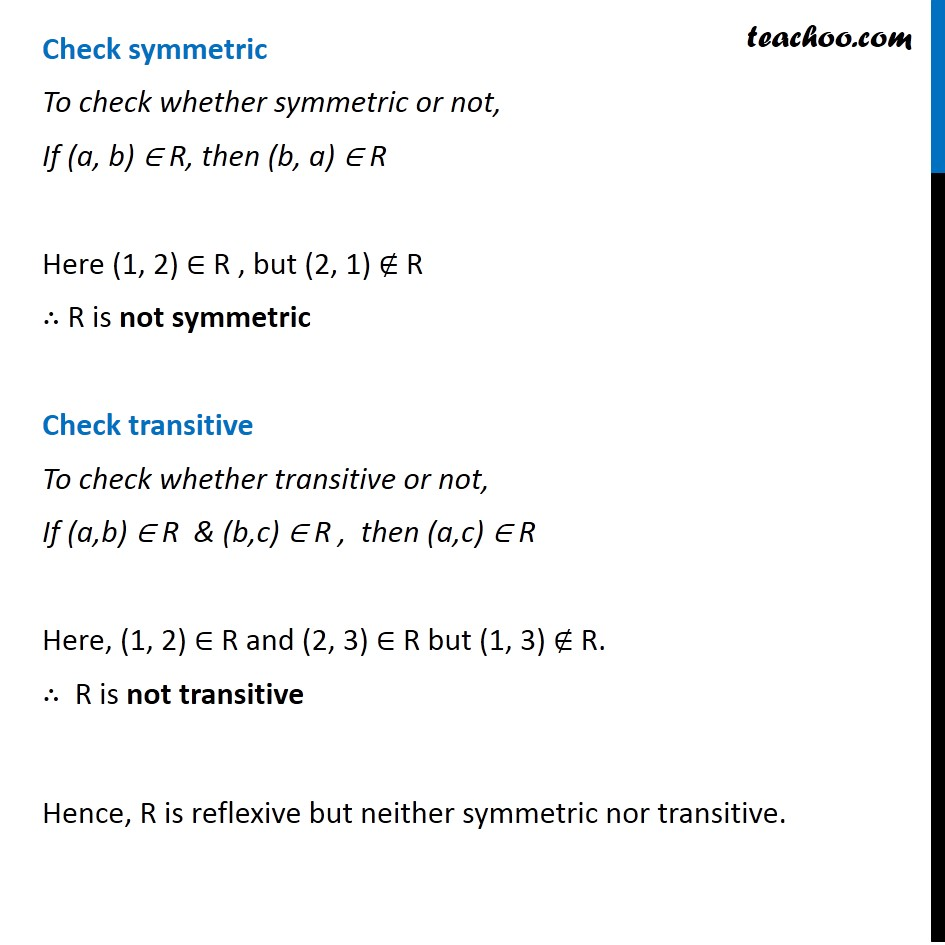Examples

Chapter 1 Class 12 Relation and Functions
Serial order wiseGet live Maths 1-on-1 Classs - Class 6 to 12

### Transcript

Example 4 Show that the relation R in the set {1, 2, 3} given by R = {(1, 1), (2, 2),(3, 3), (1, 2), (2, 3)} is reflexive but neither symmetric nor transitive. R = {(1, 1), (2, 2), (3, 3), (1, 2), (2, 3)} Check Reflexive If the relation is reflexive, then (a, a) ∈ R for every a ∈ {1, 2, 3} Since (1, 1) ∈ R ,(2, 2) ∈ R & (3, 3) ∈ R ∴ R is reflexive Check symmetric To check whether symmetric or not, If (a, b) ∈ R, then (b, a) ∈ R Here (1, 2) ∈ R , but (2, 1) ∉ R ∴ R is not symmetric Check transitive To check whether transitive or not, If (a,b) ∈ R & (b,c) ∈ R , then (a,c) ∈ R Here, (1, 2) ∈ R and (2, 3) ∈ R but (1, 3) ∉ R. ∴ R is not transitive Hence, R is reflexive but neither symmetric nor transitive.